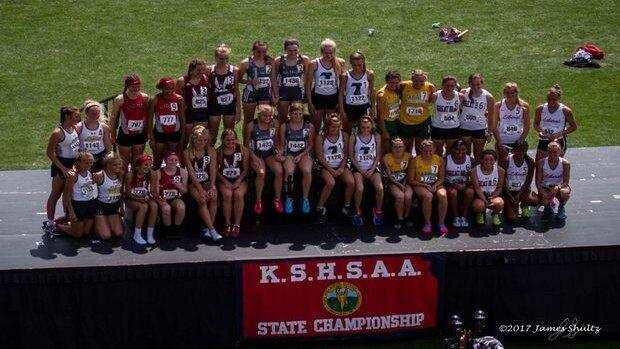# Preseason Class 4A Team Rankings

##These rankings were calculated by scoring the rankings as a meet. We took the individual rankings and relay rankings and scored them as a normal meet: 10-8-6-5-4-3-2-1.

Athletes whose graduation years are unknown were left out of the list.

Relay teams are also not included in preseason rankings

## Boys

### 80.93

Brayden Meyer (PV 9 = 9)
Braydon Gorges (110H 8 = 8)
Carson Fair (D 8 = 8)
Cole Landers (HJ 6 = 6)
Connor Hennes (400m 4 + 800m 2 = 6)
Creighton Camp (S 3 = 3)
Daniel Reichart (LJ 2 + TJ 3 = 5)
Easton Hunter (HJ 1.43 + TJ 2 = 3.43)
Eli Rowland (110H 2 = 2)
Joseph Spexarth (PV 5.5 = 5.5)
Ty McPhail (PV 9 = 9)

### 51

Bo Robison (J 4 = 4)
Connor Hasz (LJ 8 = 8)
Jake Karr (300H 3 + D 1 = 4)
Lamont Hill (LJ 3.5 + TJ 8 = 11.5)
Preston Martin (PV 5.5 = 5.5)
Rowan West (D 2 + S 5 = 7)
Ryan Wokutch (100m 1 + 200m 10 = 11)

### 41

Brady Blanka (J 8 = 8)
Caleb Shea (800m 4 = 4)
Jack Watson (100m 8 + 200m 8 + 400m 8 = 24)
Taybor Vetter (LJ 5 = 5)

### 37.5

Carson Key (LJ 1 + TJ 10 = 11)
Jake Ryan (HJ 5 = 5)
James Jackson (D 4 + S 1 = 5)
Mac Moeller (TJ 1 = 1)
Phillippe Wesley (200m 4.5 = 4.5)
Trey Ausler (110H 6 + 300H 5 = 11)

### 35.43

Brandon Snell (100m 2 + 200m 4.5 = 6.5)
David Holmes (100m 3.5 + 400m 10 = 13.5)
Jami Tyson (HJ 1.43 = 1.43)
Lajames White (LJ 6 = 6)
Owen Roellchen (1600m 3 + 3200m 5 = 8)

### 34.5

Jack Konrade (LJ 10 = 10)
Javion Lee (LJ 3.5 = 3.5)
Tanner Newkirk (1600m 10 + 3200m 8 = 18)
William Devader (J 3 = 3)

### 25

Col Bixler-large (1600m 5 + 800m 8 = 13)
Tanner Cash (300H 2 = 2)
Trace Tjaden (J 10 = 10)
7.Wellington High School25
Christian Buckman (400m 5 = 5)
Gavin York (110H 10 + 300H 10 = 20)
9.Tonganoxie High School24
Cole Sample (D 10 + S 10 = 20)
Dallas Bond (110H 4 = 4)
10.Ottawa High School21
Jayson Brown (D 6 + S 8 = 14)
Josiah Jahn (3200m 2 = 2)
Ryan Jensen (J 5 = 5)